script) -->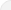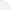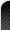# MCD Delhi Circle Rate Finder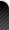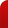## Delhi MCD Circle Rates Finder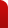The MCD (Municipal Corporation of Delhi) Rate Finder helps user to find out the current prices, per square meter, of MCD land available for sale in the Delhi region. This tool contains the information of 2311 localities within the city and gives the user the following information:

• Rate of land per square meter
• Category
• Ward Name
• Ward Number
• Zone Name
• Zone Number
All the user needs to do is click on the dropdown under the "Colony" section to find out the names of localities where MCD land is available, once a user selects the desired location, they can see the price, per square meter, at which it is available, the category, the ward name and number and the zone name and number.

This information is helpful to potential buyers who are looking at purchasing MCD Land in Delhi and covers a vast area of the city where MCD land is available for sale.

## MCD Delhi new circle rate in various localities:

All areas in Delhi have been categorized into eight types - A, B, C, D, E, F, G, and H.

December 2015 MCD Delhi circle rates are as follows:

CategoryMinimum Land Rate ( Per sq. mtr.)Minimum Flats Rate ( Per sq. mtr.)
A-1Rs. 10,00,000Rs. 5,00,000
ARs. 7,00,000Rs. 2,50,000
BRs. 4,20,000Rs. 1,50,000
CRs. 2,50,000Rs. 1,20,000
DRs. 1,50,000Rs. 80,000
ERs. 90,000Rs. 70,000
FRs. 63,000Rs. 60,000
GRs. 45,000Rs. 50,000
HRs. 30,000Rs. 40,000
Cost of Construction
CategoryMinimum Land Rate of Construction ( Per sq. mtr.)
Existing RateRecommended Rate
ARs. 18,300/-Rs. 20,130/-
BRs. 14,500/-Rs. 15,950/-
CRs. 11,600/-Rs. 12,760/-
DRs. 9,300/-Rs. 10,230/-
ERs. 7,800/-Rs. 8,580/-
FRs. 6,850/-Rs. 8,580/-
GRs. 5,800/-Rs. 8,580/-
HRs. 2,900/-Rs. 8,580/-

September 2014 MCD Delhi circle rates are as follows:
• A :: Rs. 7,75,000 Per Square Meter
• B :: Rs. 2,45,520 Per Square Meter
• C :: Rs. 1,59,840 Per Square Meter
• D :: Rs. 1,27,680 Per Square Meter
• E :: Rs. 70,070 Per Square Meter
• F :: Rs. 56,640 Per Square Meter
• G :: Rs. 46200 Per Square Meter
• H :: Rs. 23,280 Per Square Meter

November 2012 MCD Delhi circle rates were as follows:
• A :: Rs. 6,45,000 per sq meter
• B :: Rs. 2,04,600 per sq meter
• C :: Rs. 1,33,224 per sq meter
• D :: Rs. 1,06,384 per sq meter
• E :: Rs. 58,365 per sq meter
• F :: Rs. 47,140 per sq meter
• G :: Rs. 38,442 per sq meter
• H :: Rs. 19,361 per sq meter

As of November 1, 2011, the MCD Delhi circle rates applicable on these categories were as follows:
• A :: Rs. 215,000 per sq meter
• B :: Rs. 136,400 per sq meter
• C :: Rs. 109,200 per sq meter
• D :: Rs. 87,200 per sq meter
• E :: Rs. 47,840 per sq meter
• F :: Rs. 38,640 per sq meter
• G :: Rs. 31,510 per sq meter
• H :: Rs. 15,870 per sq meter
The user just has to click on the dropdown menu under the "colony" section of the rate finder to locate the names of the neighborhoods where Municipal Corporation of Delhi land is on sale. As soon as the user chooses the preferred area, he can view the rate of per sq meter, name of the ward, the type of land as well as the zone number and name.

Last Updated on: November 03, 2020